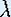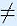Next: Logistic Growth Model
Prev: Introduction

# One-Dimensional Dynamical Systems

## Warm Up Questions

For these questions, you should first read the Introduction. We start with an exploration of linear dynamical systems. Later on you will learn how (easy) linear systems help to explain the dynamics of (complicated) nonlinear systems.

Let us consider the linear dynamical system

f(x) =x

as it was introduced in the Introduction. For now, do not think in terms of squirrels too much, both x andcan be negative numbers, and we accept non-integer values for them as well.

1. The graph of f is simply a straight line. Draw the graphs for= 0.1, 0.3, 1, 2, and 5. Explain for eachwhat the long term behavior of the system is for arbitrary x. What happens for x = 0? What happens for= 1?

Now look at the slope of each graph. Do you see a relation between the long term behavior of the system and the slope of its graph?

2. For negativethe graph of f is still a straight line. Draw the graphs for= -3, -1.8, -1, -0.5, and -0.2. For eachdescribe the long term behavior, and explain the difference in behavior compared to positive.

Formulate the relation between the long term behavior of the system and the slope of its graph, for both positive and negative slopes.

3. In the above two exercises you have found two special lines, corresponding to two special linear systems, namely f(x) = x and f(x) = -x. Draw the graphs of these two functions. In the same picture sketch the graph of a nonlinear function which is such that iterates of this function go to infinity for all x0.

Make another picture with the graph of a nonlinear function whose iterates go to 0 for all x.

To summarize the results:

The iterates of a linear system either go to 0 or to infinity, for all x0.

More complicated dynamics can only be obtained with nonlinear dynamical systems. You should already be able to guess what the graph of such a function needs to look like, in order to get different dynamics. Let us consider nonlinear dynamical stystems that can be found on your calculator.

4. Suppose we have a dynamical system given by the square root function on your calculator. Type in any number and calculate its square root. Take the square root of the result. Take the square root again and so on. Give a full description of the dynamics, similar to what was done for the squirrel model. In particular, look for values of x that do not change when taking the square root. Only consider x0.

Draw the graph of the square root function, together with the two special lines y = x and y = -x. What is special about the points where the graph of the square root function intersects one of the two lines?

5. Give a description of the dynamics for a dynamical system given by the cosine button, where x is given in radians.

Draw a graph of the cosine function for x given in radians. Also draw the special lines y = x and y = -x. Describe what is special about the points on the intersection of the graph of the cosine function and these two lines.

Next: Logistic Growth ModelThe Geometry Center Home Page